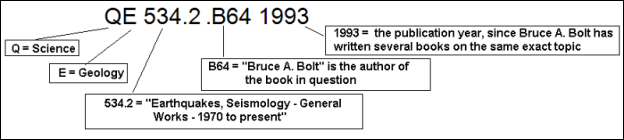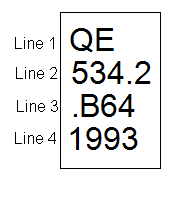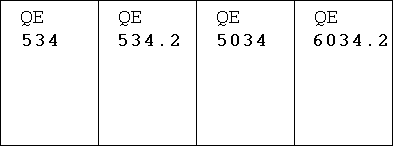## Answered By: Colin Magee Last Updated: Jan 15, 2019     Views: 972

The Loman C. Trover library uses the Library of Congress Classification System to organize its books.

Library of Congress Classification System is an alpha-numeric system.  Books are arranged by broad academic subjects, then by more specific subjects, and then by the author's name and year.

Call numbers

Each book in the library has a unique call number. The call number tells you the book's location on the shelf, just like your house number tells you your house's location on your street.

Each LCC call number is represented by a combination of capital letters and numbers

Let's take a look at the following book and its call number. The book is called Earthquakes by Bruce A. Bolt, published in 1993.Call number: QE 534.2 .B64 1993

The first part of any call number is a pair of capital letters (or sometimes a single capital letter): in this case, QE.

 QE 534.2 .B64 1993

The first capital letter in the sequence always represents one of the twenty-one areas of broad knowledge.
In this example, Q = "Science"

The second capital letter indicates a subclass (a smaller division of the twenty-one areas of broad knowledge).
In this example, E= "Geology," which is a smaller division under the broader heading of "Science."

So, we already know that the book in question has to do with GEOLOGY, based on the two capital letters, and that all books that have to do with Geology will always begin with QE.

The next thing following the capital letters is a string of numbers. The first string of numbers represents a specific aspect within the subclass.

 QE 534.2 .B64 1993

We already know that anything beginning with QE has to do with GEOLOGY, but all of the books on GEOLOGY still have to be organized based on specific aspects of GEOLOGY. So, any book with a call number ranging from QE 500 to QE 625 are books that have to do with "Dynamic and Structural Geology."  In our example, 534.2 is the specific number within the QE 500 to QE 635 range assigned to books within "Dynamic and Structural Geology" that have to do with "Earthquakes, Seismology – General Works – 1970 to Present."

Following the capital letters and the first set of numbers is a decimal point, which then contains a single capital letter and a second string of numbers.

 QE 534.2 .B64 1993

This part of the call number distinguishes between all the books that have been written on the very same subject – in this case, all the books on "Earthquakes, Seismology – General Works – 1970 to Present." This part of the call number is called the Cutter Number.

In this example, the Cutter Number is .B64.  This specific number was chosen because the book in our example was written by an author named Bruce A. Bolt.  B is for "Bolt," and 64 is to distinguish it from all the other books on Earthquakes that were written by authors whose last names also begin with the letter B.

Since Bruce A. Bolt has written several books on Earthquakes, the book needs to be further distinguished.  Therefore, the date of publication is the final thing added to the call number, in this case, 1993.

This diagram shows you all the components of the call number and what they represent in our example.Order of books on the shelf

When a call number is physically placed on a book so it can go on the shelf, the call number of a book gets broken into three or four separate lines and placed on the spine of the book.The LCC System organizes materials in alpha-numeric order, line by line.

Line 1. The books are first sorted by the first line, which is the capital letters which represent the main class and the subclass. When shelved, single letters are arranged first.  Double letters follow single letters.  The letters below are in correct order from left to right:Line 2. Materials that have the exact same first line, like QE for example, are then shelved in order by their second line: the numbers which followed the initial one or two capital letters.  Remember that these numbers represent a more-specific aspect of the main class & subclass. Whole numbers are sorted first, and then by the numbers following the decimal point.  The call numbers below are in correct order from left to right.Line 3. Line three usually begins with a decimal point followed by the Cutter Number

Materials that contain the same first and second lines are then shelved by the third line, first alphabetically by the capital letter, and then digit-by-digit following that letter.  The numbers which follow the capital letter in this line are in decimal form -- they are not whole numbers.  Therefore, numbers in Line 3 are filed digit by digit rather than by all of their digits at once.  (This is the part of the call number that is by far the most confusing.)

For example, .B64 is shelved before .B7, because the first digit in .B64 is a 6, and the first digit in .B7 is a 7.  It doesn't matter that .B64 has a second digit and .B7 doesn't. We're only interested in the first digit, because those are the first digits following the capital letter which differ from one another.

If they have the same first digit, then you look at the second digit. So the number .B765 is filed before .B77. Since they have the same first digit (7), you move onto the second digit. The second digit in .B765 is 6, and the second digit in .B77 is 7, and 6 comes before 7.  Now you would stop here. It doesn't matter that .B765 has a third digit and .B77 doesn't, because as soon as a digit differs between the two call numbers, you sort them based on that digit, and then you stop.

If you become confused, simply imagine an extra zero on the end of the one number which is shorter, to make it the same length as the one that's longer. So for example, when comparing .B64 to .B7, you can imagine an extra zero exists on .B7 to make it .B70. Now you're comparing .B64 to .B70. It's easier to see which one comes first, now. In the second example, .B77 becomes .B770 when you compare it to a number with three digits like .B765. It's easier to see that .B765 comes before .B770 now.

The call numbers below are in correct order from left to right.Line 4. Many call numbers are only three lines long.  Sometimes there will be a second Cutter Number added to the call number, which would follow the same pattern as line 3 as it is actually a continuation of the third line that began with a decimal point.  Although this fourth line will not begin with a decimal point, the numbers are still decimals -- not whole numbers. These numbers are filed like those in line three, digit by digit. The call numbers below are in correct order from left to right.If the call number has only three lines (and no second Cutter Number), this fourth line will usually be the date of publication (i.e., 2003).  If the preceding lines are all identical, then the items will be shelved in chronological order by the date of publication.  The call numbers below are in correct order from left to right.